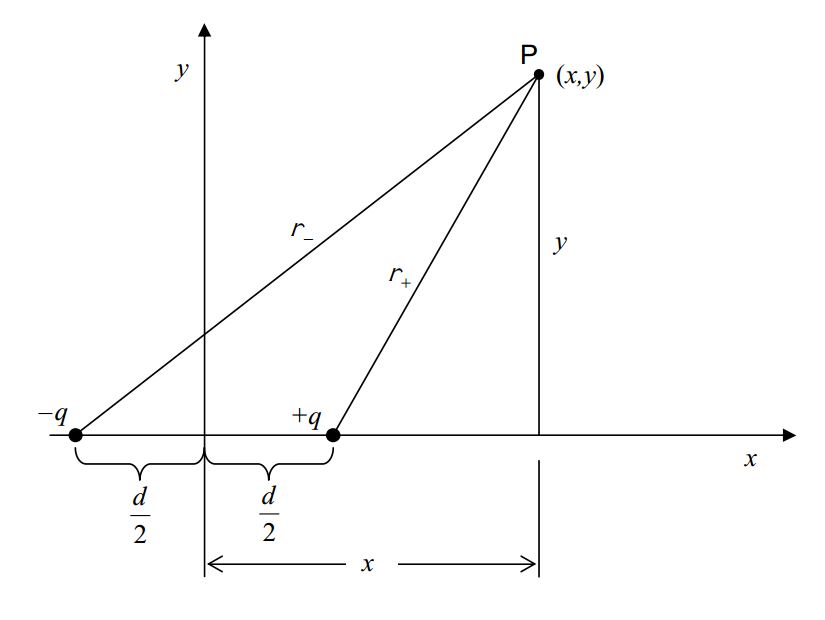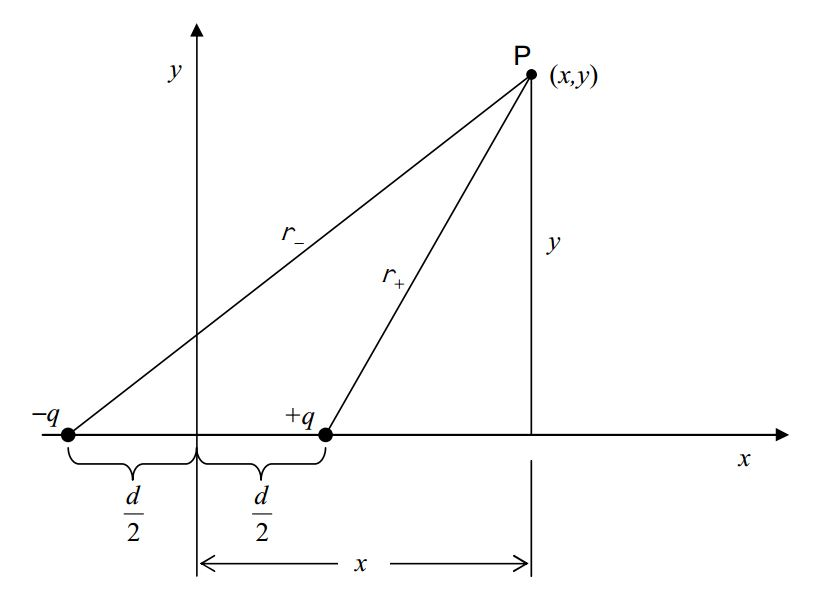# B6: The Electric Potential Due to One or More Point Charges

$$\newcommand{\vecs}{\overset { \rightharpoonup} {\mathbf{#1}} }$$ $$\newcommand{\vecd}{\overset{-\!-\!\rightharpoonup}{\vphantom{a}\smash {#1}}}$$$$\newcommand{\id}{\mathrm{id}}$$ $$\newcommand{\Span}{\mathrm{span}}$$ $$\newcommand{\kernel}{\mathrm{null}\,}$$ $$\newcommand{\range}{\mathrm{range}\,}$$ $$\newcommand{\RealPart}{\mathrm{Re}}$$ $$\newcommand{\ImaginaryPart}{\mathrm{Im}}$$ $$\newcommand{\Argument}{\mathrm{Arg}}$$ $$\newcommand{\norm}{\| #1 \|}$$ $$\newcommand{\inner}{\langle #1, #2 \rangle}$$ $$\newcommand{\Span}{\mathrm{span}}$$ $$\newcommand{\id}{\mathrm{id}}$$ $$\newcommand{\Span}{\mathrm{span}}$$ $$\newcommand{\kernel}{\mathrm{null}\,}$$ $$\newcommand{\range}{\mathrm{range}\,}$$ $$\newcommand{\RealPart}{\mathrm{Re}}$$ $$\newcommand{\ImaginaryPart}{\mathrm{Im}}$$ $$\newcommand{\Argument}{\mathrm{Arg}}$$ $$\newcommand{\norm}{\| #1 \|}$$ $$\newcommand{\inner}{\langle #1, #2 \rangle}$$ $$\newcommand{\Span}{\mathrm{span}}$$$$\newcommand{\AA}{\unicode[.8,0]{x212B}}$$

The electric potential due to a point charge is given by

$\varphi=\frac{kq}{r}\label{6-1}$

where

• $$\varphi$$ is the electric potential due to the point charge,
• $$k=8.99\times 10^9 \frac{Nm^2}{C^2}$$ is the Coulomb constant,
• $$q$$ is the charge of the particle (the source charge, a.k.a. the point charge) causing the electric field for which the electric potential applies, and,
• $$r$$ is the distance that the point of interest is from the point charge.

In the case of a non-uniform electric field (such as the electric field due to a point charge), the electric potential method for calculating the work done on a charged particle is much easier than direct application of the force-along-the-path times the length of the path. Suppose, for instance, a particle of charge $$q′$$ is fixed at the origin and we need to find the work done by the electric field of that particle on a victim of charge $$q$$ as the victim moves along the $$x$$ axis from $$x_1$$ to $$x_2$$. We can’t simply calculate the work as

$F\cdot(x_2-x_1) \nonumber$

even though the force is in the same direction as the displacement, because the force $$F$$ takes on a different value at every different point on the $$x$$ axis from $$x = x_1$$ to $$x = x_2$$. So, we need to do an integral:

$dW=Fdx \nonumber$

$dW=qEdx \nonumber$

$dW=q\frac{kq'}{x^2} dx \nonumber$

$\int dW=\int_{x_1}^{x_2} q\frac{kq'}{x^2} dx \nonumber$

$W=kq'q\int_{x_1}^{x_2} x^{-2} dx \nonumber$

$W=kq'q \frac{x^{-1}}{-1}\Big |_{x_1}^{x_2} \nonumber$

$W=-kq'q(\frac{1}{x_2}-\frac{1}{x_1}) \nonumber$

$W=-(\frac{kq'q}{x_2}-\frac{kq'q}{x_1}) \nonumber$

Compare this with the following solution to the same problem (a particle of charge $$q′$$ is fixed at the origin and we need to find the work done by the electric field of that particle on a victim of charge $$q$$ as the victim moves along the $$x$$ axis from $$x_1$$ to $$x_2$$):

$W=-\Delta U \nonumber$

$W=-q \Delta \varphi \nonumber$

$W=-q(\varphi_2-\varphi_1) \nonumber$

$W=-(\frac{kq'q}{x_2}-\frac{kq'q}{x_1}) \nonumber$

The electric potential energy of a particle, used in conjunction with the principle of the conservation of mechanical energy, is a powerful problem-solving tool. The following example makes this evident:

A particle of charge 0.180 $$\mu C$$ is fixed in space by unspecified means. A particle of charge -0.0950 $$\mu C$$ and mass 0.130 grams is 0.885 cm away from the first particle and moving directly away from the first particle with a speed of 15.0 m/s. How far away from the first particle does the second particle get?

This is a conservation of energy problem. As required for all conservation of energy problems, we start with a before and after diagram:$$\mbox{Energy Before = Energy After}$$

$K+U=K'+U' \nonumber$

$K+q\varphi=0+q \varphi' \nonumber$

$\frac{1}{2}mv^2+q\frac{kq_s}{r}=q\frac{kq_s}{r'} \nonumber$

$\frac{1}{r'}=\frac{1}{r}+\frac{mv^2}{2kq_sq} \nonumber$

$r'=\frac{1}{\frac{1}{r}+\frac{mv^2}{2kq_sq}} \nonumber$

$r'=\frac{1}{ \frac{1}{8.85\times 10^{-3} m} + \frac{1.30\times 10^{-4}kg(15.0 m/s)^2}{2(8.99\times 10^9 \frac{N\cdot m^2}{C^2})1.80\times 10^{-7}C(-9.50\times 10^{-8}C) } } \nonumber$

$r'=0.05599m \nonumber$

$r'=0.0560m \nonumber$

$r'=5.6cm \nonumber$

## Superposition in the Case of the Electric Potential

When there is more than one charged particle contributing to the electric potential at a point in space, the electric potential at that point is the sum of the contributions due to the individual charged particles. The electric potential at a point in space, due to a set of several charged particles, is easier to calculate than the electric field due to the same set of charged particles is. This is true because the sum of electric potential contributions is an ordinary arithmetic sum, whereas, the sum of electric field contributions is a vector sum.

Find a formula that gives the electric potential at any point $$(x, y)$$ on the x-y plane, due to a pair of particles: one of charge $$–q$$ at $$(-\frac{d}{2},0)$$ and the other of charge $$+q$$ at $$(\frac{d}{2},0)$$.

Solution

We establish a point $$P$$ at an arbitrary position $$(x, y)$$ on the x-y plain and determine the distance that point $$P$$ is from each of the charged particles. In the following diagram, I use the symbol $$r_{+}$$ to represent the distance that point $$P$$ is from the positively-charged particle, and $$r_{-}$$ to represent the distance that point P is from the negatively-charged particle.Analysis of the shaded triangle in the diagram at right gives us $$r_{+}$$.

$r_{+}=\sqrt{(x-\frac{d}{2})^2+y^2} \nonumber$Analysis of the shaded triangle in the diagram at right gives us $$r_{-}$$.

$r_{-}=\sqrt{(x+\frac{d}{2})^2+y^2} \nonumber$With the distances that point $$P$$ is from each of the charged particles in hand, we are ready to determine the potential:

$r_{+}=\sqrt{(x-\frac{d}{2})^2+y^2} \nonumber$

$r_{-}=\sqrt{(x+\frac{d}{2})^2+y^2} \nonumber$$\varphi(x,y)=\varphi_{+}+\varphi_{-} \nonumber$

$\varphi(x,y)=\frac{kq}{r_{+}}+\frac{k(-q)}{r_{-}} \nonumber$

$\varphi(x,y)=\frac{kq}{r_{+}}-\frac{kq}{r_{-}} \nonumber$

$\varphi(x,y)=\frac{kq}{\sqrt{(x-\frac{d}{2})^2+y^2}}-\frac{kq}{\sqrt{(x+\frac{d}{2})^2+y^2}} \nonumber$

This page titled B6: The Electric Potential Due to One or More Point Charges is shared under a CC BY-SA 2.5 license and was authored, remixed, and/or curated by Jeffrey W. Schnick via source content that was edited to the style and standards of the LibreTexts platform; a detailed edit history is available upon request.